# Selina Concise Mathematics Class 8 ICSE Solutions Chapter 23 Probability

## Selina Concise Mathematics Class 8 ICSE Solutions Chapter 23 Probability

Selina Publishers Concise Mathematics Class 8 ICSE Solutions Chapter 23 Probability

### Probability Exercise 23 – Selina Concise Mathematics Class 8 ICSE Solutions

Question 1.
A die is thrown, find the probability of getting:
(i) a prime number
(ii) a number greater than 4
(iii) a number not greater than 4.
Solution:Question 2.
A coin is tossed. What is the probability of getting:
Solution: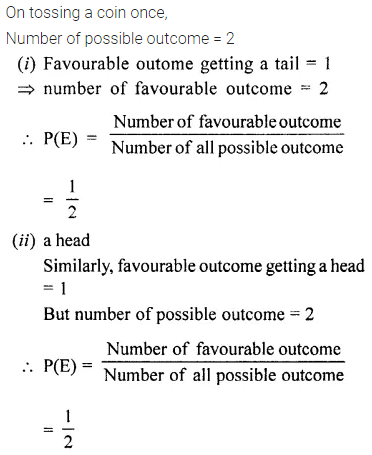Question 3.
A coin is tossed twice. Find the probability of getting:
(i) exactly one head (ii) exactly one tail
(iii) two tails (iv) two heads
Solution: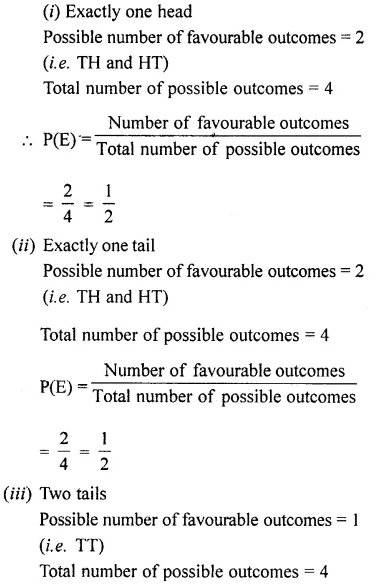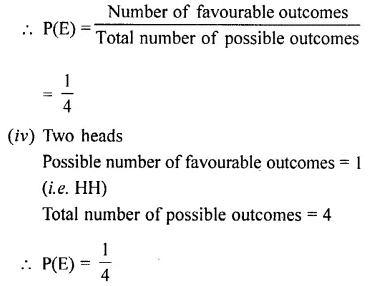Question 4.
A letter is chosen from the word ‘PENCIL’ what is the probability that the letter chosen is a consonant?
Solution: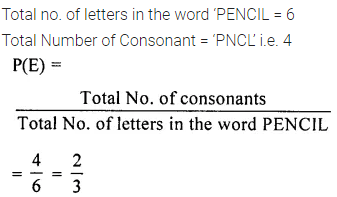Question 5.
A bag contains a black ball, a red ball and a green ball, all the balls are identical in shape and size. A ball is drawn from the bag without looking into it. What is the probability that the ball drawn is:
(i) a red ball
(ii) not a red ball
(iii) a white ball.
Solution:Question 6.
6. In a single throw of a die, find the probability of getting a number
(i) greater than 2
(ii) less than or equal to 2
(iii) not greater than 2.
Solution: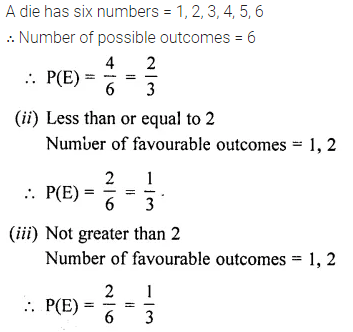Question 7.
A bag contains 3 white, 5 black and 2 red balls, all of the same shape and size.
A ball is drawn from the bag without looking into it, find the probability that the ball drawn is:
(i) a black ball.
(ii) a red ball.
(iii) a white ball.
(iv) not a red ball.
(v) not a black ball.
Solution: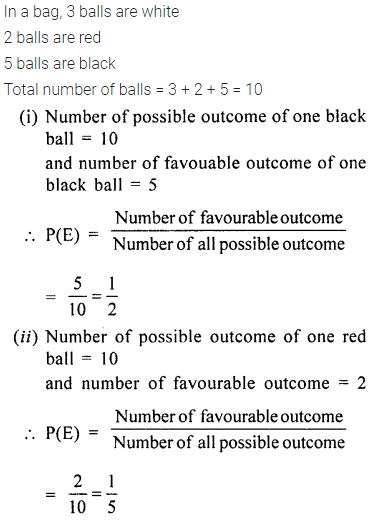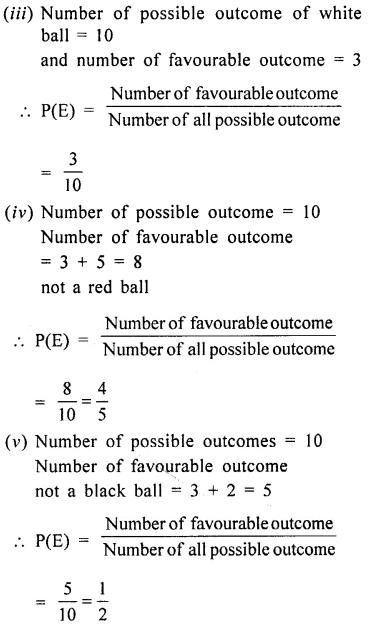Question 8.
In a single throw of a die, find the probability that the number:
(i) will be an even number.
(ii) will be an odd number.
(iii) will not be an even number.
Solution: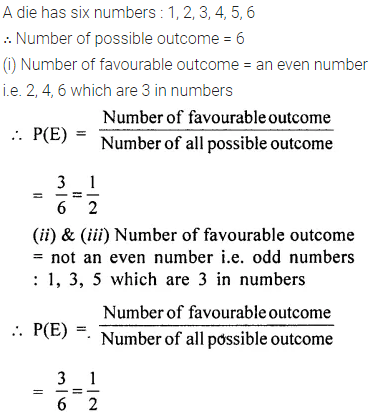Question 9.
In a single throw of a die, find the probability of getting :
(i) 8
(ii) a number greater than 8
(iii) a number less than 8
Solution: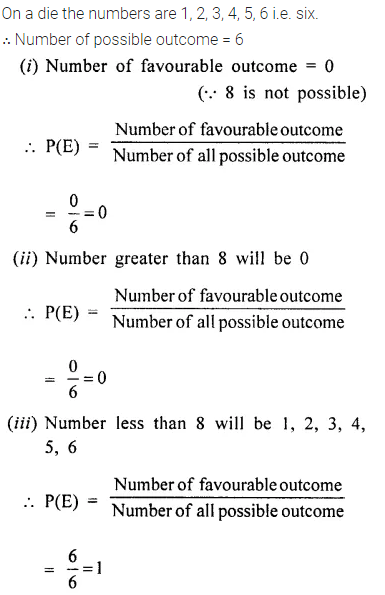Question 10.
Which of the following can not be the probability of an event?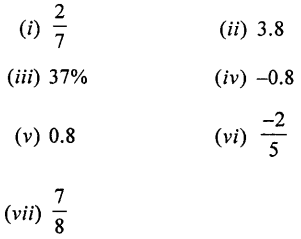Solution:Question 11.
A bag contains six identical black balls. A child withdraws one ball from the bag without looking into it. What is the probability that he takes out:
(i) a white ball,
(ii) a black ball
Solution:Question 12.
Three identical coins are tossed together. What is the probability of obtaining:
Solution:Question 13.
A book contains 92 pages. A page is chosen at random. What is the probability that the sum of the digits in the page number is 9?
Solution: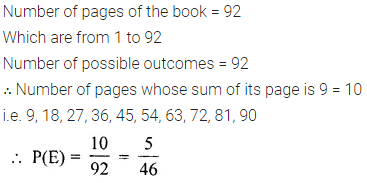Question 14.
Two coins are tossed together. What is the probability of getting:
(ii) both heads or both tails.
Solution:Question 15.
From 10 identical cards, numbered 1, 2, 3, …… , 10, one card is drawn at random. Find the probability that the number on the card drawn is a multiple of:
(i) 2 (ii) 3
(iii) 2 and 3 (iv) 2 or 3
Solution:Question 16.
Two dice are thrown at the same time. Find the probability that the sum of the two numbers appearing on the top of the dice is:
(i) 0
(ii) 12
(iii) less than 12
(iv) less than or equal to 12
Solution:Question 17.
A die is thrown once. Find the probability of getting:
(i) a prime number
(ii) a number greater than 3
(iii) a number other than 3 and 5
(iv) a number less than 6
(v) a number greater than 6.
Solution:Question 18.
Two coins are tossed together. Find the probability of getting:
(i) exactly one tail